## UltraScale Architecture Clocking Resources User Guide (UG572)

Document ID
UG572
Release Date
2023-02-01
Revision
1.10.2 English

Spread-spectrum clock generation (SSCG) is widely used by manufacturers of electronic devices to reduce the spectral density of the electromagnetic interference (EMI) generated by these devices. Manufacturers must ensure that levels of electromagnetic energy emitted do not interfere with the operation of other nearby electronic devices. For example, the clarity of a phone call should not degrade when the phone is next to a video display. In the same way, the display should not be affected when the phone is used.

Electromagnetic compatibility (EMC) regulations are used to control the noise or EMI that causes these disturbances. Typical solutions for meeting EMC requirements involve adding expensive shielding, ferrite beads, or chokes. These solutions can adversely impact the cost of the final product by complicating PCB routing and forcing longer product development cycles.

SSCG spreads the electromagnetic energy over a large frequency band to effectively reduce the electrical and magnetic field strengths measured within a narrow window of frequencies. The peak electromagnetic energy at any one frequency is reduced by modulating the SSCG output.

The MMCME# can generate a spread-spectrum clock from a standard fixed frequency oscillator when SS_EN is set to TRUE (see This Figure ). Within the MMCME#, the VCO frequency is modulated along with CLKFBOUT and CLKOUT[6:4,1,0]. Clock outputs CLKOUT[3:2] are used to control the modulation period and are not available for general use. As long as the clock frequency is adjusted slowly, the spread-spectrum does not affect the period jitter of the MMCME#.

 X-Ref Target - Figure 3-12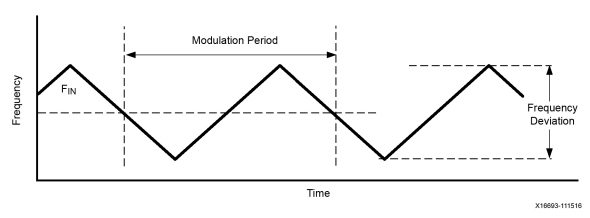Adjusting the modulation period SS_MOD_PERIOD allows you to direct the tools to select the closest modulation period based on the MMCME# settings. The spread-spectrum modulation reduces EMI as long as the modulation frequencies are higher than the audible frequency range of 30 KHz. Typically, lower modulation frequencies are preferred to minimize the impact of the introduction of spread-spectrum.

Increasing the frequency deviation with SS_MODE (CENTER_HIGH or DOWN_HIGH) increases the overall EMI reduction, but care must be taken to ensure that the increased range of frequencies does not affect the overall system operation (see This Figure ). Because the spread-spectrum clock and the input clock are operating at different frequencies, any data being transferred between the clock domains should use an asynchronous FIFO to ensure that data is not lost. Increasing the frequency deviation requires a larger FIFO.

 X-Ref Target - Figure 3-13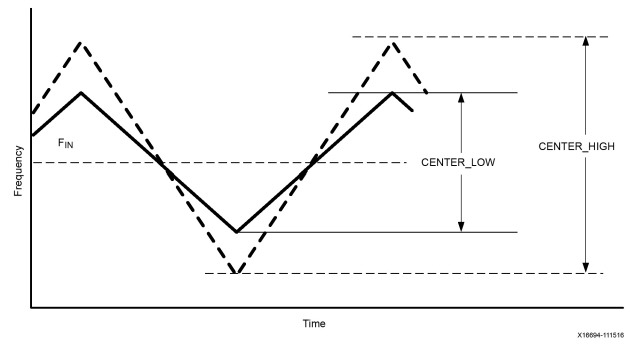Another design trade-off is the decision to use a center spread or down spread. Selecting SS_MODE (DOWN_HIGH, DOWN_LOW) spreads the frequencies to lower frequencies as shown in This Figure . DOWN_HIGH has similar frequency deviation to CENTER_LOW.

 X-Ref Target - Figure 3-14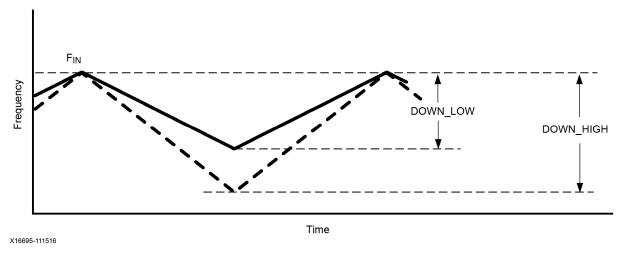The decision to use down spread is often the result of considering the timing analysis impact of spread-spectrum. When using a spread-spectrum clock, the design must meet timing at the highest frequency in the frequency deviation. Therefore, if a 100 MHz clock with SS_MODE (CENTER_LOW) produces a 3% (±1.5%) center spread, the 100 MHz clock with 3% center spread must pass timing analysis as a 101.5 MHz clock. However, if SS_MODE (DOWN_HIGH) produces a 3% down spread, the input frequency is the highest frequency within the frequency deviation. Consequently, for a 100 MHz clock with 3% down spread, the down-spread clock would continue to be analyzed by timing analysis as a 100 MHz clock.

Table 3-5: Manual SS Timing Adjustment Using Input Frequency for UltraScale Devices

Parameter

Input Frequency (MHz)

M

SS_MODE(CENTER_HIGH)

25 < F IN < 35

M = 28

FIN_SS = F IN x 56/55

35 < F IN < 50

M = 21

FIN_SS = F IN x 42/41

M = 22

FIN_SS = FIN x 44/43

50 < F IN < 75

M = 28

FIN_SS = F IN x 56/55

75 < F IN < 150

M = 21

FIN_SS = F IN x 42/41

M = 22

FIN_SS = F IN x 44/43

SS_MODE (CENTER_LOW)

25 < F IN < 35

M = 56

FIN_SS = F IN x 112/111

35 < F IN < 50

M = 42

FIN_SS = F IN x 84/83

M= 44

FIN_SS = F IN x 88/87

50 < F IN < 75

M = 56

FIN_SS = F IN x 112/111

75 < F IN < 150

M = 42

FIN_SS = F IN x 84/83

M = 44

FIN_SS = F IN x 88/87

SS_MODE (DOWN_HIGH)

25 < F IN < 35

M=28

FIN_SS = F IN

35 < F IN < 50

M = 21, 22

FIN_SS = F IN

50 < F IN < 75

M = 28

FIN_SS = F IN

75 < F IN < 100

M = 21, 22

FIN_SS = F IN

100 < F IN < 150

M = 21, 22

FIN_SS = F IN

SS_MODE (DOWN_LOW)

25 < F IN < 35

M = 56

FIN_SS = F IN

35 < F IN < 50

M = 42, 44

FIN_SS = F IN

50 < F IN < 75

M = 56

FIN_SS = F IN

75 < F IN < 100

M = 42, 44

FIN_SS = F IN

100 < F IN < 150

M = 42, 44

FIN_SS = F IN

Table 3-6: Manual SS Timing Adjustment Using Input Frequency for UltraScale+ Devices

Parameter

Input Frequency (MHz)

M

D

SS_MODE (CENTER_HIGH)

30 < F IN < 40

M = 28

D = 1

FIN_SS = F IN x 56/55

40 < F IN < 60

M = 21

D = 1

FIN_SS = F IN x 42/41

M = 22

D = 1

FIN_SS = FIN x 44/43

60 < F IN < 80

M = 28

D = 2

FIN_SS = F IN x 56/55

80 < F IN < 120

M = 21

D = 2

FIN_SS = F IN x 42/41

M = 22

D = 2

FIN_SS = F IN x 44/43

120 < F IN < 150

M = 21

D = 3

FIN_SS = F IN x 42/41

M = 22

D = 3

FIN_SS = F IN x 44/43

SS_MODE (CENTER_LOW)

30 < F IN < 40

M = 56

D = 2

FIN_SS = F IN x 112/111

40 < F IN < 60

M = 42

D = 2

FIN_SS = F IN x 84/83

M = 44

D = 2

FIN_SS = F IN x 88/87

60 < F IN < 80

M = 56

D = 4

FIN_SS = F IN x 112/111

80 < F IN < 120

M = 42

D = 4

FIN_SS = F IN x 84/83

M = 44

D = 4

FIN_SS = F IN x 88/87

120 < F IN < 150

M = 42

D = 6

FIN_SS = F IN x 84/83

M = 44

D = 6

FIN_SS = F IN x 88/87

SS_MODE (DOWN_HIGH)

35 < F IN < 40

M=28

D = 1

FIN_SS = F IN

40 < F IN < 60

M = 21, 22

D = 1

FIN_SS = F IN

60 < F IN < 80

M = 28

D = 2

FIN_SS = F IN

80 < F IN < 120

M = 21, 22

D = 2

FIN_SS = F IN

120 < F IN < 150

M = 21, 22

D = 3

FIN_SS = F IN

SS_MODE (DOWN_LOW)

35 < F IN < 40

M = 56

D = 2

FIN_SS = F IN

40 < F IN < 60

M = 42, 44

D = 2

FIN_SS = F IN

60 < F IN < 80

M = 56

D = 4

FIN_SS = F IN

80 < F IN < 120

M = 42, 44

D = 4

FIN_SS = F IN

120 < F IN < 150

M = 42, 44

D = 6

FIN_SS = F IN

For a 25 MHz input clock, the new timing constraints would be:

SS_MODE(CENTER_HIGH) = 25 x 56/55 = 25.45 MHz

SS_MODE (CENTER_LOW) = 25 x 112/111 = 25.23 MHz

SS_MODE (DOWN_HIGH) = 25 MHz

SS_MODE (DOWN_LOW) = 25 MHz

For an 80 MHz input clock, the new timing constraints would be:

SS_MODE(CENTER_HIGH) = 80 x 44/43 = 81.86 MHz

SS_MODE (CENTER_LOW) = 80 x 88/87 = 80.92 MHz

SS_MODE (DOWN_HIGH) = 80 MHz

SS_MODE (DOWN_LOW) = 80 MHz

Table: Manual SS Timing Adjustment Using Input Frequency for UltraScale Devices and Table: Manual SS Timing Adjustment Using Input Frequency for UltraScale+ Devices provide information which allows the manual adjustment of timing constraints to the frequency range of the spread-spectrum enabled clock. This is for the generation of timing constraints in an XDC file used by Vivado tools.

Table: Manual SS Timing Adjustment Using Input Frequency for UltraScale Devices and Table: Manual SS Timing Adjustment Using Input Frequency for UltraScale+ Devices show that timing constraints should be modified when spread-spectrum clocking parameter SS_MODE is set to CENTER_LOW or CENTER_HIGH. When SS_MODE attribute is set to DOWN_LOW or DOWN_HIGH timing constraint adjustment is not necessary.

Also note that manually adjusting timing constraints is not needed because the Vivado tools detect when spread-spectrum clocking in a design. Vivado tools (static timing analysis) automatically account for any timing spread caused by the spread-spectrum enabled clocks. When spread-spectrum clocks are used, Vivado static timing analysis adds a spread-spectrum (SS) uncertainty value of the total uncertainty calculation formula. The formula used by the static analysis tools is as follows:

Equation 3-12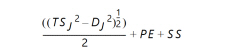where:

TS J 2 = Total system jitter

D J 2 = Discrete jitter

PE = Phase error

CAUTION! When using spread-spectrum clocking in a design, it is necessary to use appropriated clock domain crossing (CDC) circuitry for all signals, data and non-data, crossing clock and spread-spectrum clock domains, and vice versa.

Asynchronous FIFOs should be used to transfer data between two clock domains. The depth of the FIFO depends on the modulation frequency in the clock. The slower the modulation, the deeper the FIFO needs to be:

Equation 3-13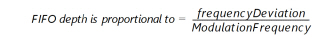When spread-spectrum clocking is used with SS_MODE set as DOWN_LOW or DOWN_HIGH the calculated F~IN_SS~ frequency (using data from Table: Manual SS Timing Adjustment Using Input Frequency for UltraScale Devices and/or Table: Manual SS Timing Adjustment Using Input Frequency for UltraScale+ Devices ) is lower than the original clock frequency (Refer to the examples after Table: Manual SS Timing Adjustment Using Input Frequency for UltraScale+ Devices ). If no precautions are taken, the used FIFO can fill up and over-run. Prevent this by using a FIFO with throttle control.

Table 3-7: Spread-Spectrum Generation Restrictions for UltraScale Devices

Parameter

Value

F MODULATION

Minimum

25 [KHz]

Maximum

250 [KHz]

Input Clock Frequency

Minimum

25 [MHz]

Maximum

150 [MHz]

SS_MODE (CENTER_HIGH)

25 MHz < F IN < 35 MHz

M = 28
D = 1

35 MHz < F IN < 50 MHz

M = 21, 22
D = 1

50 MHz < F IN < 75 MHz

M = 28
D = 2

75 MHz < F IN < 100 MHz

M = 21, 22
D = 2

100 MHz < F IN < 150 MHz

M = 21, 22
D = 3

SS_MODE (CENTER_LOW)

25 MHz < F IN < 35 MHz

M = 56
D = 2

35 MHz < F IN < 50 MHz

M = 42, 44
D = 2

50 MHz < F IN < 75 MHz

M = 56
D = 4

75 MHz < F IN < 100 MHz

M = 42, 44
D = 4

100 MHz < F IN < 150 MHz

M = 42, 44
D = 6

SS_MODE (DOWN_HIGH)

25 MHz < F IN < 35 MHz

M = 28
D = 1

35 < F IN < 50 MHz

M = 21, 22
D = 1

50 MHz < F IN < 75 MHz

M = 28
D = 2

75 MHz < F IN < 100 MHz

M = 21, 22
D = 2

100 MHz < F IN < 150 MHz

M = 21, 22
D = 3

SS_MODE (DOWN_LOW)

25 MHz < F IN < 35 MHz

M = 56
D = 2

35 MHz < F IN < 50 MHz

M = 42, 44
D = 2

50 MHz < F IN < 75 MHz

M = 56
D = 4

75 MHz < F IN < 100 MHz

M = 42, 44
D = 4

100 MHz < F IN < 150 MHz

M = 42, 44
D = 6

CLKOUT[3:2]_DIVIDE

N/A

CLKOUT[6:4,1,0]_DIVIDE

1 to 128

Bandwidth

Low

Table 3-8: Spread-Spectrum Generation Restrictions for UltraScale+ Devices

Parameter

Value

F MODULATION

Minimum

30 [KHz]

Maximum

250 [KHz]

Input Clock Frequency

Minimum

25 [MHz]

Maximum

150 [MHz]

SS_MODE (CENTER_HIGH)

30 MHz < F IN < 40 MHz

M = 28
D = 1

40 MHz < F IN < 60 MHz

M = 21, 22
D = 1

60 MHz < F IN < 80 MHz

M = 28
D = 2

80 MHz < F IN < 120 MHz

M = 21, 22
D = 2

120 MHz < F IN < 150 MHz

M = 21, 22
D = 3

SS_MODE (CENTER_LOW)

30 MHz < F IN < 40 MHz

M = 56
D = 2

40 MHz < F IN < 60 MHz

M = 42, 44
D = 2

60 MHz < F IN < 80 MHz

M = 56
D = 4

80 MHz < F IN < 120 MHz

M = 42, 44
D = 4

120 MHz < F IN < 150 MHz

M = 42, 44
D = 6

SS_MODE (DOWN_HIGH)

30 MHz < F IN < 40 MHz

M = 28
D = 1

40 MHz < F IN < 60 MHz

M = 21, 22
D = 1

60 MHz < F IN < 80 MHz

M = 28
D = 2

80 MHz < F IN < 120 MHz

M = 21, 22
D = 2

120 MHz < F IN < 150 MHz

M = 21, 22
D = 3

SS_MODE (DOWN_LOW)

30 MHz < F IN < 40 MHz

M = 56
D = 2

40 MHz < F IN < 60 MHz

M = 42, 44
D = 2

60 MHz < F IN < 80 MHz

M = 56
D = 4

80 MHz < F IN < 120 MHz

M = 42, 44
D = 4

120 MHz < F IN < 150 MHz

M = 42, 44
D = 6

CLKOUT[3:2]_DIVIDE

N/A

CLKOUT[6:4,1,0]_DIVIDE

1 to 128

Bandwidth

Low

When using spread-spectrum generation, the VCO frequency is set by the clocking wizard based on the input frequency and SS_MODE. As a result, the clocking wizard is recommended to set the output frequencies for CLKOUT[6:4,1,0].

Based on the VCO frequency and SS_MOD_PERIOD, the clocking wizard also determines the correct modulation settings to set the modulation frequency within 10% of SS_MOD_PERIOD. Because the modulation frequency is dependent on the VCO frequency, the modulation frequency scales as the input frequency changes for a given compilation.